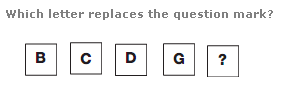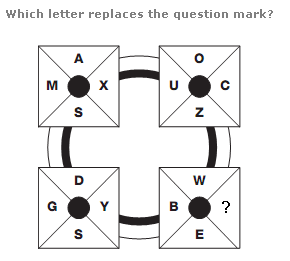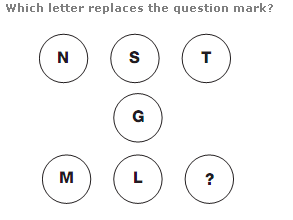# Puzzles - Missing letters puzzles

### Exercise :: Missing letters puzzlesAnswer : J Explanation : Working from top to bottom, letters appear in alphabetical order, skipping any letters written with only straight lines.Answer : Q Explanation : Starting with the top left square, and moving clockwise around the other squares, the numerical values of each letter increase by 2, with their relative positions in each square rotating 1 place clockwise at each turn.Answer : G Explanation : Starting with the letters in the top row, and following straight lines through the middle circle, subtract the numerical value of the middle letter (G) and write the letter with the corresponding numerical value in the lower circle.